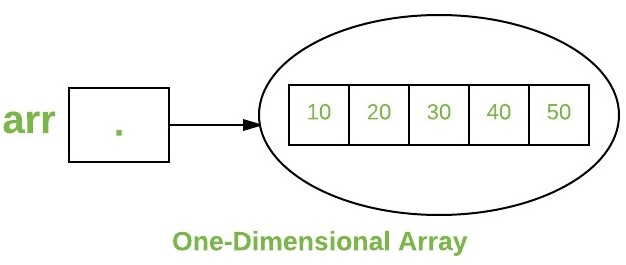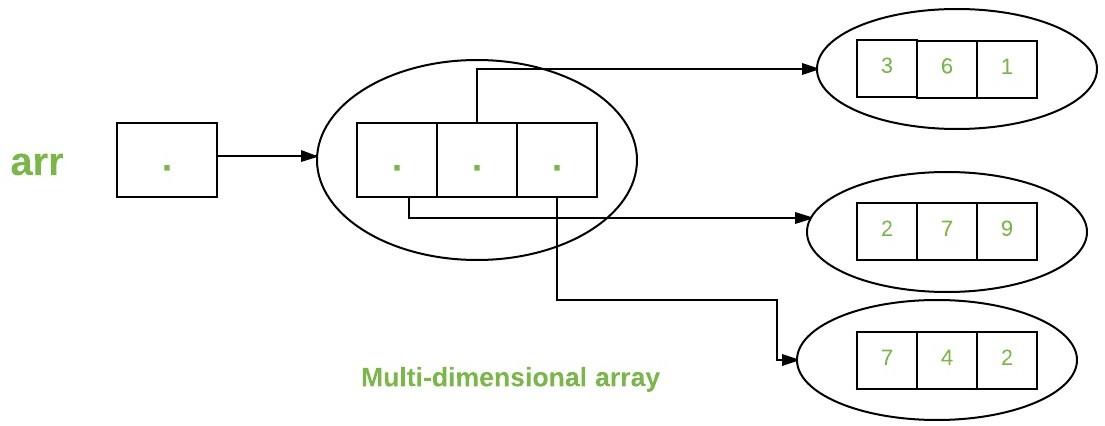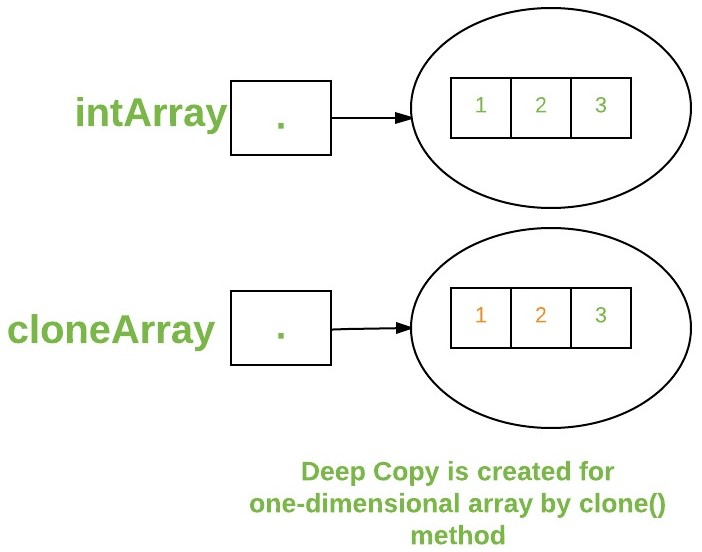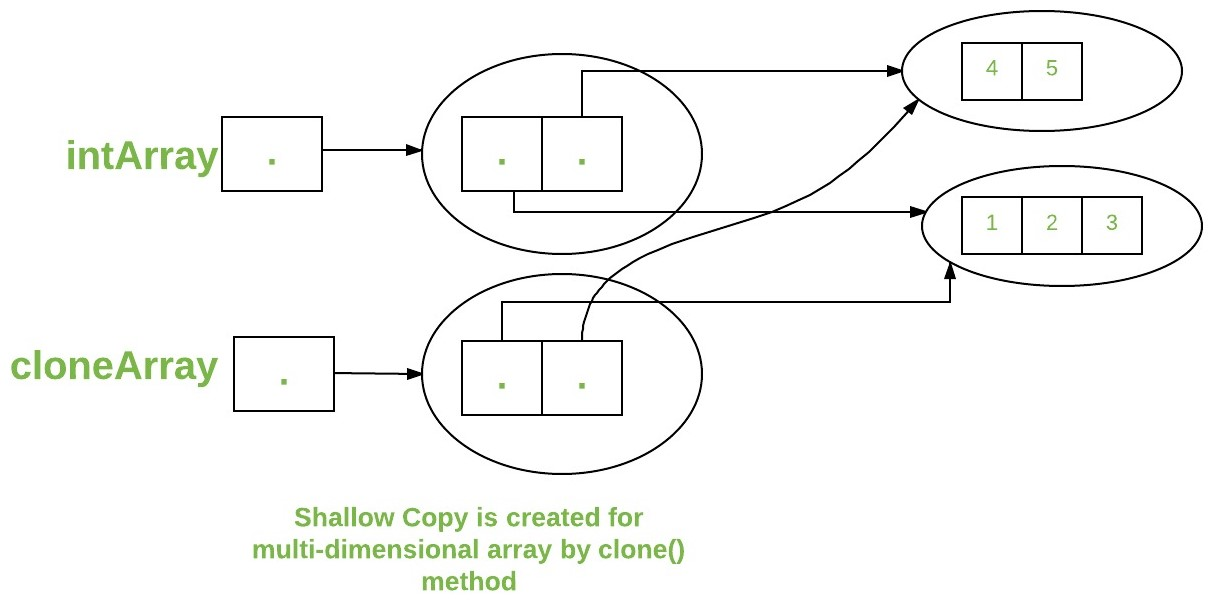# Arrays in Java

• Difficulty Level : Easy
• Last Updated : 08 Jul, 2022

An array in Java is a group of like-typed variables referred to by a common name. Arrays in Java work differently than they do in C/C++. Following are some important points about Java arrays.

• In Java, all arrays are dynamically allocated. (discussed below)
• Arrays are stored in contagious memory [consecutive memory locations].
• Since arrays are objects in Java, we can find their length using the object property length. This is different from C/C++, where we find length using sizeof.
• A Java array variable can also be declared like other variables with [] after the data type.
• The variables in the array are ordered, and each has an index beginning from 0.
• Java array can also be used as a static field, a local variable, or a method parameter.
• The size of an array must be specified by int or short value and not long.
• The direct superclass of an array type is Object.
• Every array type implements the interfaces Cloneable and java.io.Serializable
• This storage of arrays helps us in randomly accessing the elements of an array [Support Random Access].
• The size of the array cannot be altered(once initialized).  However, an array reference can be made to point to another array.

An array can contain primitives (int, char, etc.) and object (or non-primitive) references of a class depending on the definition of the array. In the case of primitive data types, the actual values are stored in contiguous memory locations. In the case of class objects, the actual objects are stored in a heap segment### One-Dimensional Arrays:

The general form of a one-dimensional array declaration is

```type var-name[];
OR
type[] var-name;```

An array declaration has two components: the type and the name. type declares the element type of the array. The element type determines the data type of each element that comprises the array. Like an array of integers, we can also create an array of other primitive data types like char, float, double, etc., or user-defined data types (objects of a class). Thus, the element type for the array determines what type of data the array will hold.

Example:

```// both are valid declarations
int intArray[];
or int[] intArray;

byte byteArray[];
short shortsArray[];
boolean booleanArray[];
long longArray[];
float floatArray[];
double doubleArray[];
char charArray[];

// an array of references to objects of
// the class MyClass (a class created by
// user)
MyClass myClassArray[];

Object[]  ao,        // array of Object
Collection[] ca;  // array of Collection
// of unknown type```

Although the first declaration establishes that intArray is an array variable, no actual array exists. It merely tells the compiler that this variable (intArray) will hold an array of the integer type. To link intArray with an actual, physical array of integers, you must allocate one using new and assign it to intArray.### Instantiating an Array in Java

When an array is declared, only a reference of an array is created. To create or give memory to the array, you create an array like this: The general form of new as it applies to one-dimensional arrays appears as follows:

`var-name = new type [size];`

Here, type specifies the type of data being allocated, size determines the number of elements in the array, and var-name is the name of the array variable that is linked to the array. To use new to allocate an array, you must specify the type and number of elements to allocate.

Example:

```int intArray[];    //declaring array
intArray = new int;  // allocating memory to array```

OR

`int[] intArray = new int; // combining both statements in one`

Note :

1. The elements in the array allocated by new will automatically be initialized to zero (for numeric types), false (for boolean), or null (for reference types). Do refer to default array values in Java.
2. Obtaining an array is a two-step process. First, you must declare a variable of the desired array type. Second, you must allocate the memory to hold the array, using new, and assign it to the array variable. Thus, in Java, all arrays are dynamically allocated.

### Array Literal

In a situation where the size of the array and variables of the array are already known, array literals can be used.

``` int[] intArray = new int[]{ 1,2,3,4,5,6,7,8,9,10 };
// Declaring array literal```
• The length of this array determines the length of the created array.
• There is no need to write the new int[] part in the latest versions of Java.

### Accessing Java Array Elements using for Loop

Each element in the array is accessed via its index. The index begins with 0 and ends at (total array size)-1. All the elements of array can be accessed using Java for Loop.

``` // accessing the elements of the specified array
for (int i = 0; i < arr.length; i++)
System.out.println("Element at index " + i +
" : "+ arr[i]);```

Implementation:

## Java

 `// Java program to illustrate creating an array``// of integers,  puts some values in the array,``// and prints each value to standard output.`` ` `class` `GFG {``    ``public` `static` `void` `main(String[] args)``    ``{``        ``// declares an Array of integers.``        ``int``[] arr;`` ` `        ``// allocating memory for 5 integers.``        ``arr = ``new` `int``[``5``];`` ` `        ``// initialize the first elements of the array``        ``arr[``0``] = ``10``;`` ` `        ``// initialize the second elements of the array``        ``arr[``1``] = ``20``;`` ` `        ``// so on...``        ``arr[``2``] = ``30``;``        ``arr[``3``] = ``40``;``        ``arr[``4``] = ``50``;`` ` `        ``// accessing the elements of the specified array``        ``for` `(``int` `i = ``0``; i < arr.length; i++)``            ``System.out.println(``"Element at index "` `+ i``                               ``+ ``" : "` `+ arr[i]);``    ``}``}`

Output

```Element at index 0 : 10
Element at index 1 : 20
Element at index 2 : 30
Element at index 3 : 40
Element at index 4 : 50```

Time Complexity: O(n)
Auxiliary Space : O(1)

You can also access java arrays using for each loops### Arrays of Objects

An array of objects is created like an array of primitive type data items in the following way.

`Student[] arr = new Student; //student is a user-defined class`

The studentArray contains seven memory spaces each of the size of student class in which the address of seven Student objects can be stored. The Student objects have to be instantiated using the constructor of the Student class, and their references should be assigned to the array elements in the following way.

`Student[] arr = new Student;`

Example

## Java

 `// Java program to illustrate creating``//  an array of objects`` ` `class` `Student {``    ``public` `int` `roll_no;``    ``public` `String name;``    ``Student(``int` `roll_no, String name)``    ``{``        ``this``.roll_no = roll_no;``        ``this``.name = name;``    ``}``}`` ` `// Elements of the array are objects of a class Student.``public` `class` `GFG {``    ``public` `static` `void` `main(String[] args)``    ``{``        ``// declares an Array of integers.``        ``Student[] arr;`` ` `        ``// allocating memory for 5 objects of type Student.``        ``arr = ``new` `Student[``5``];`` ` `        ``// initialize the first elements of the array``        ``arr[``0``] = ``new` `Student(``1``, ``"aman"``);`` ` `        ``// initialize the second elements of the array``        ``arr[``1``] = ``new` `Student(``2``, ``"vaibhav"``);`` ` `        ``// so on...``        ``arr[``2``] = ``new` `Student(``3``, ``"shikar"``);``        ``arr[``3``] = ``new` `Student(``4``, ``"dharmesh"``);``        ``arr[``4``] = ``new` `Student(``5``, ``"mohit"``);`` ` `        ``// accessing the elements of the specified array``        ``for` `(``int` `i = ``0``; i < arr.length; i++)``            ``System.out.println(``"Element at "` `+ i + ``" : "``                               ``+ arr[i].roll_no + ``" "``                               ``+ arr[i].name);``    ``}``}`

Output

```Element at 0 : 1 aman
Element at 1 : 2 vaibhav
Element at 2 : 3 shikar
Element at 3 : 4 dharmesh
Element at 4 : 5 mohit```

Time Complexity: O(n)
Auxiliary Space : O(1)

### Example

An array of objects is also created like an array of primitive type data items.

`Student[] studentArray; // No array created`
`Student[] studentArray = new Student; // Array of 5 students created but No students are there in the array`

The studentArray contains five memory spaces each of the size of the student class in which the address of seven Student objects can be stored. The Student objects have to be instantiated using the constructor of the Student class, and their references should be assigned to the array elements in the following way.

## Java

 `// Java program to illustrate creating``//  an array of objects``   ` `class` `Student``{``    ` `    ``public` `String name;``    ``Student(String name)``    ``{``        ``this``.name = name;``    ``}``      ``@Override``    ``public` `String toString(){``        ``return` `name;``    ``}``}``   ` `// Elements of the array are objects of a class Student.``public` `class` `GFG``{``    ``public` `static` `void` `main (String[] args)``    ``{``           ``// declares an Array and initializing  the elements of the array``        ``Student[] myStudents = ``new` `Student[]{``new` `Student(``"Dharma"``),``new` `Student(``"sanvi"``),``new` `Student(``"Rupa"``),``new` `Student(``"Ajay"``)}; ``// Array of 5 students created but No students are there in the array``   ` `        ``// accessing the elements of the specified array``        ``for``(Student m:myStudents){    ``            ``System.out.println(m);``        ``}``    ``}``}`

Output

```Dharma
sanvi
Rupa
Ajay```

### What happens if we try to access elements outside the array size?

JVM throws ArrayIndexOutOfBoundsException to indicate that the array has been accessed with an illegal index. The index is either negative or greater than or equal to the size of an array.

## Java

 `public` `class` `GFG {``    ``public` `static` `void` `main(String[] args)``    ``{``        ``int``[] arr = ``new` `int``[``2``];``        ``arr[``0``] = ``10``;``        ``arr[``1``] = ``20``;`` ` `        ``for` `(``int` `i = ``0``; i < arr.length; i++)``            ``System.out.println(arr[i]);``    ``}``}`

Output

```10
20```

### Multidimensional Arrays:

Multidimensional arrays are arrays of arrays with each element of the array holding the reference of other arrays. These are also known as Jagged Arrays. A multidimensional array is created by appending one set of square brackets ([]) per dimension.```int[][] intArray = new int; //a 2D array or matrix
int[][][] intArray = new int; //a 3D array```

Example

## Java

 `public` `class` `multiDimensional {``    ``public` `static` `void` `main(String args[])``    ``{``        ``// declaring and initializing 2D array``        ``int` `arr[][]``            ``= { { ``2``, ``7``, ``9` `}, { ``3``, ``6``, ``1` `}, { ``7``, ``4``, ``2` `} };`` ` `        ``// printing 2D array``        ``for` `(``int` `i = ``0``; i < ``3``; i++) {``            ``for` `(``int` `j = ``0``; j < ``3``; j++)``                ``System.out.print(arr[i][j] + ``" "``);`` ` `            ``System.out.println();``        ``}``    ``}``}`

Output

```2 7 9
3 6 1
7 4 2 ```

### Passing Arrays to Methods

Like variables, we can also pass arrays to methods. For example, the below program passes the array to method sum to calculate the sum of the array’s values.

## Java

 `// Java program to demonstrate``// passing of array to method`` ` `public` `class` `Test {``    ``// Driver method``    ``public` `static` `void` `main(String args[])``    ``{``        ``int` `arr[] = { ``3``, ``1``, ``2``, ``5``, ``4` `};`` ` `        ``// passing array to method m1``        ``sum(arr);``    ``}`` ` `    ``public` `static` `void` `sum(``int``[] arr)``    ``{``        ``// getting sum of array values``        ``int` `sum = ``0``;`` ` `        ``for` `(``int` `i = ``0``; i < arr.length; i++)``            ``sum += arr[i];`` ` `        ``System.out.println(``"sum of array values : "` `+ sum);``    ``}``}`

Output

`sum of array values : 15`

Time Complexity: O(n)

Auxiliary Space : O(1)

### Returning Arrays from Methods

As usual, a method can also return an array. For example, the below program returns an array from method m1

## Java

 `// Java program to demonstrate``// return of array from method`` ` `class` `Test {``    ``// Driver method``    ``public` `static` `void` `main(String args[])``    ``{``        ``int` `arr[] = m1();`` ` `        ``for` `(``int` `i = ``0``; i < arr.length; i++)``            ``System.out.print(arr[i] + ``" "``);``    ``}`` ` `    ``public` `static` `int``[] m1()``    ``{``        ``// returning  array``        ``return` `new` `int``[] { ``1``, ``2``, ``3` `};``    ``}``}`

Output

`1 2 3 `

Time Complexity: O(n),
Auxiliary Space : O(1)

### Class Objects for Arrays

Every array has an associated Class object, shared with all other arrays with the same component type.

## Java

 `// Java program to demonstrate``// Class Objects for Arrays`` ` `class` `Test {``    ``public` `static` `void` `main(String args[])``    ``{``        ``int` `intArray[] = ``new` `int``[``3``];``        ``byte` `byteArray[] = ``new` `byte``[``3``];``        ``short` `shortsArray[] = ``new` `short``[``3``];`` ` `        ``// array of Strings``        ``String[] strArray = ``new` `String[``3``];`` ` `        ``System.out.println(intArray.getClass());``        ``System.out.println(``            ``intArray.getClass().getSuperclass());``        ``System.out.println(byteArray.getClass());``        ``System.out.println(shortsArray.getClass());``        ``System.out.println(strArray.getClass());``    ``}``}`

Output

```class [I
class java.lang.Object
class [B
class [S
class [Ljava.lang.String;```

Explanation:

1. The string “[I” is the run-time type signature for the class object “array with component type int.”
2. The only direct super class of an array type is java.lang.Object.
3. The string “[B” is the run-time type signature for the class object “array with component type byte.”
4. The string “[S” is the run-time type signature for the class object “array with component type short.”
5. The string “[L” is the run-time type signature for the class object “array with component type of a Class.” The Class name is then followed.

### Array Members

Now, as you know that arrays are objects of a class, and a direct superclass of arrays is a class Object. The members of an array type are all of the following:

• The public final field length, which contains the number of components of the array. Length may be positive or zero.
• All the members inherited from class Object; the only method of Object that is not inherited is its clone method.
• The public method clone(), which overrides the clone method in class Object and throws no checked exceptions.

### Cloning of arrays

When you clone a single-dimensional array, such as Object[], a “deep copy” is performed with the new array containing copies of the original array’s elements as opposed to references.

## Java

 `// Java program to demonstrate``// cloning of one-dimensional arrays`` ` `class` `Test {``    ``public` `static` `void` `main(String args[])``    ``{``        ``int` `intArray[] = { ``1``, ``2``, ``3` `};`` ` `        ``int` `cloneArray[] = intArray.clone();`` ` `        ``// will print false as deep copy is created``        ``// for one-dimensional array``        ``System.out.println(intArray == cloneArray);`` ` `        ``for` `(``int` `i = ``0``; i < cloneArray.length; i++) {``            ``System.out.print(cloneArray[i] + ``" "``);``        ``}``    ``}``}`

Output

```false
1 2 3 ```A clone of a multi-dimensional array (like Object[][]) is a “shallow copy,” however, which is to say that it creates only a single new array with each element array a reference to an original element array, but subarrays are shared.

## Java

 `// Java program to demonstrate``// cloning of multi-dimensional arrays`` ` `class` `Test {``    ``public` `static` `void` `main(String args[])``    ``{``        ``int` `intArray[][] = { { ``1``, ``2``, ``3` `}, { ``4``, ``5` `} };`` ` `        ``int` `cloneArray[][] = intArray.clone();`` ` `        ``// will print false``        ``System.out.println(intArray == cloneArray);`` ` `        ``// will print true as shallow copy is created``        ``// i.e. sub-arrays are shared``        ``System.out.println(intArray[``0``] == cloneArray[``0``]);``        ``System.out.println(intArray[``1``] == cloneArray[``1``]);``    ``}``}`

Output

```false
true
true```Related Articles:

This article is contributed by Nitsdheerendra and Gaurav Miglani. If you like GeeksforGeeks and would like to contribute, you can also write an article using write.geeksforgeeks.org or mail your article to review-team@geeksforgeeks.org. See your article appearing on the GeeksforGeeks main page and help other Geeks.

My Personal Notes arrow_drop_up